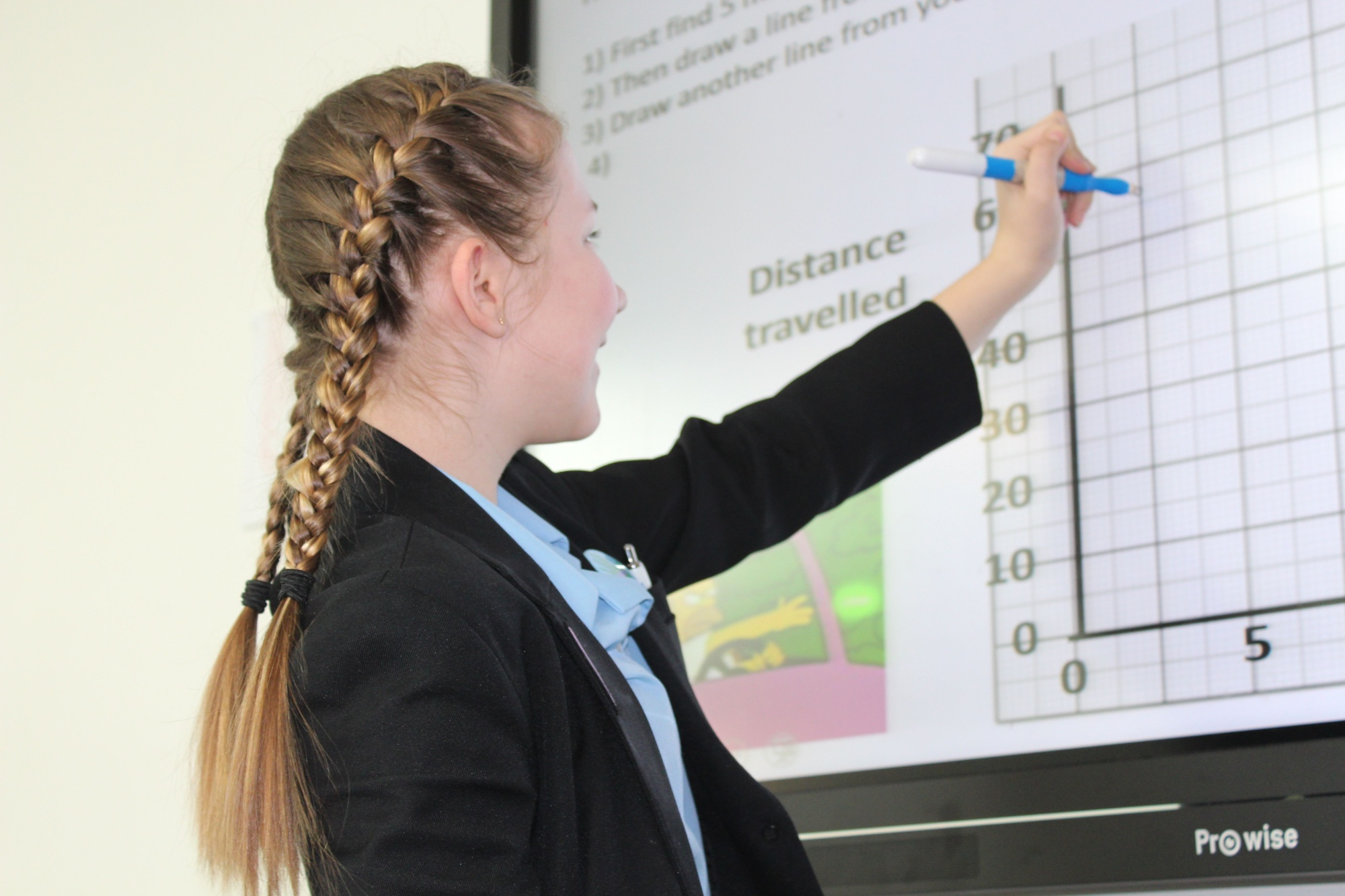# St John’s Catholic Comprehensive SchoolYou Are Here:

# Maths

Year 7 is our first opportunity to instil a love of Maths into our students. All students up until this point have had very different experiences of Mathematics; we try to expose our students to a Mathematical world of problems and puzzles as well as providing a good foundation for their years leading into GCSE study.In Year 8 we build on this and start to think about preparing our students for GCSE topics.

Year 7

Term 1

Analysing and Displaying Data – students use different types of graphs and averages.

Number Properties – students look at different types of number and how to use them

Term 2

Fractions – students learn how to compare and simplify fractions.

Probability – students will look at the language of probability and how to calculate theoretical and experimental probability.

Term 3

Decimals and Measure – students work with rounding decimals and look at different units of measurement, including area and perimeter.

Term 4

Ratio and Proportion – students work on simplifying ratios and sharing in given ratios and proportions.

Term 5

Lines and Angles – students start work on construction and mathematical drawing

Sequences and Graphs – students start with basic patterns and move on to pure numerical sequences where algebra is started to be applied.

Term 6

Expressions, Functions and Formulae – students now start to look at algebra and how the abstract can lend itself to the concrete Maths they are used to.

Assessment

All students have a termly ‘Milestone’ test.

This test checks everything they have been taught up to and including that term; this ensures that students are given constant reminders of earlier topics, giving them the best chance at retention.

All students then complete an End of Year Test, covering topics taught that academic year.

Year 8

Term 1

Number – students look at negative numbers, powers, roots, multiples and factors.

Statistics, Graphs and Charts – students move on from Year 7 graphs to now look at pie charts, stem and leaf diagrams, scatter graphs and their application.

Term 2

Transformation – students look at the movement of shapes.

Expressions and Equations – students learn how to manipulate algebra including expanding and factorising brackets and solving equations.

Term 3

Calculating with fractions – students use the four operations with fractions, and start to compare fractions, decimals and percentages.

Decimals and Ratio – students build on Year 7 work on ratio and proportion by introducing decimals.

Term 4

Probability – students move on from traditional probability to now use diagrams to represent probability problems.

Percentages, Decimals and Fractions – students compare and use fractions, decimals and percentages.

Term 5

Multiplicative reasoning – students look at real world application of percentages

Real-life graphs – students look at graphs and statistics used in the real-world including conversion graphs and distance-time graphs.

Term 6

Construction- students use mathematical equipment to construct different shapes.

Circles – students look at the different measurements associated with circles.

Assessment

All students have a termly ‘Milestone’ test.

This test checks everything they have been taught up to and including that term; this ensures that students are given constant reminders of earlier topics, giving them the best chance at retention.

All students then complete an End of Year Test, covering topics taught that academic year.

What will I gain from Studying Maths?

KS3 Maths is the foundation of studying GCSE Maths.

Students should also leave KS3 with a keen diligence to solve problems, an attribute much desired in other subjects.

Pathways this course can lead to

All students must study GCSE Maths, but the best outcomes in KS3 can lead to studying Higher GCSE content.

Extra curricular opportunities

Chess Club

Drop-in sessions